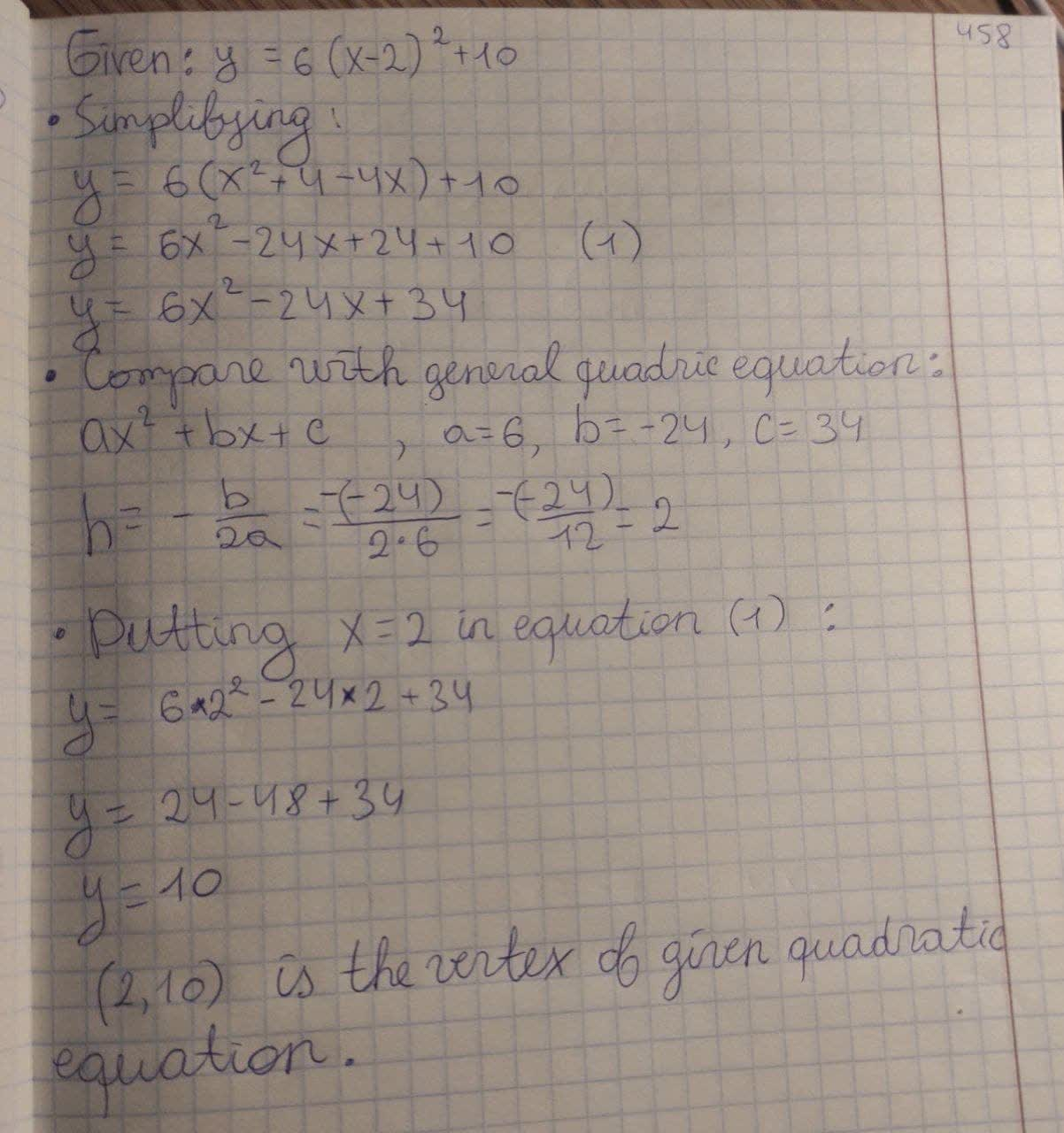Mylo O'Moore

2021-02-22

What is the vertex of the quadratic $y=6\left(x-2{\right)}^{2}+10$?Latisha Oneil

$y=6{\left(x-2\right)}^{2}+10$
On simplifying,
$y=6\left({x}^{2}+4-4x\right)+10$
$y=6{x}^{2}-24x+24+10$ (1)
$y=6{x}^{2}-24x+34$
Now, this equation compare with general quadratic equation,
$a{x}^{2}+bx+c$
a = 6 , b = -24 , c = 34
$h=-\frac{b}{2}a$
$=-\frac{-24}{2\cdot 6}$
= 2
Now putting x= 2 in equation (i), then we get
$y=6\cdot {2}^{2}-24\cdot 2+34$
y = 24 - 48 + 34
y = 10
(2, 10) is the vertex of given quadratic equation.Do you have a similar question?